# An Introduction to Classical Logic propositional and Predicate

• Slides: 51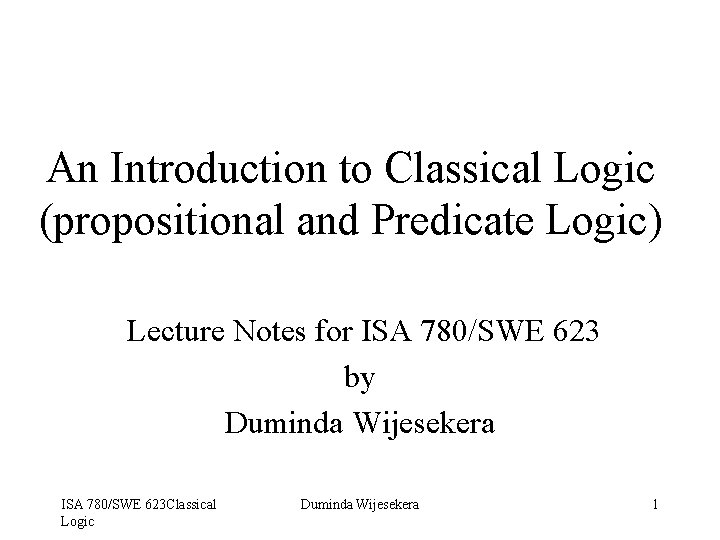An Introduction to Classical Logic (propositional and Predicate Logic) Lecture Notes for ISA 780/SWE 623 by Duminda Wijesekera ISA 780/SWE 623 Classical Logic Duminda Wijesekera 1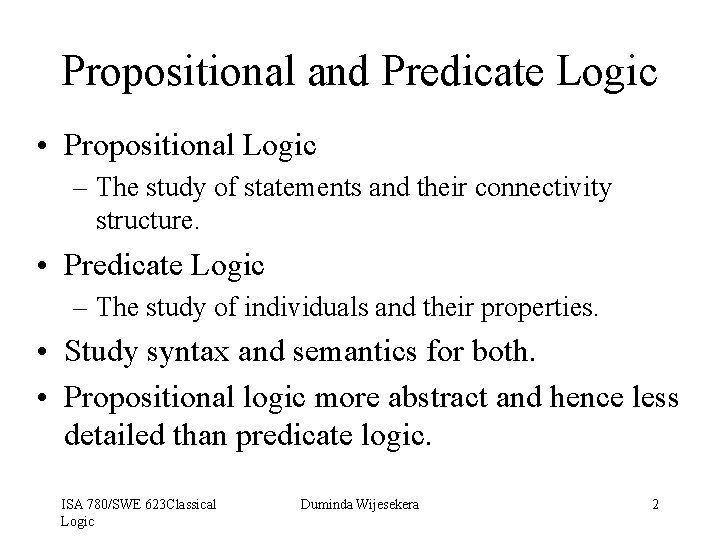Propositional and Predicate Logic • Propositional Logic – The study of statements and their connectivity structure. • Predicate Logic – The study of individuals and their properties. • Study syntax and semantics for both. • Propositional logic more abstract and hence less detailed than predicate logic. ISA 780/SWE 623 Classical Logic Duminda Wijesekera 2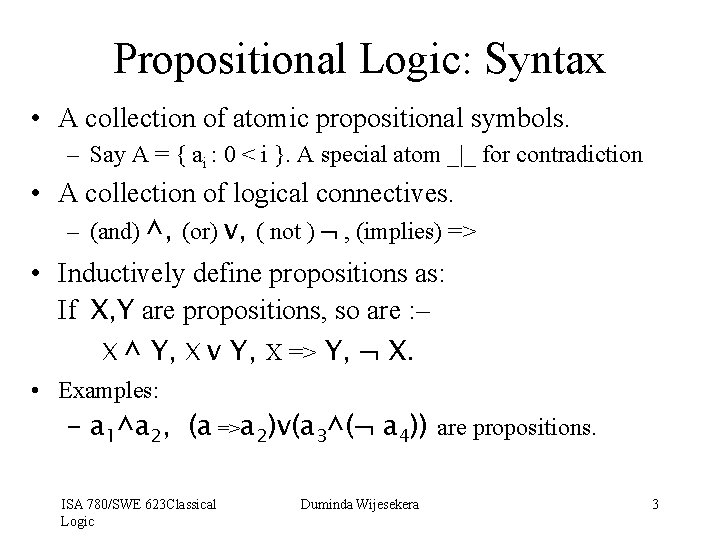Propositional Logic: Syntax • A collection of atomic propositional symbols. – Say A = { ai : 0 < i }. A special atom _|_ for contradiction • A collection of logical connectives. – (and) ^, (or) v, ( not ) , (implies) => • Inductively define propositions as: If X, Y are propositions, so are : – X ^ Y, X v Y, X => Y, X. • Examples: – a 1^a 2, (a =>a 2)v(a 3^( a 4)) are propositions. ISA 780/SWE 623 Classical Logic Duminda Wijesekera 3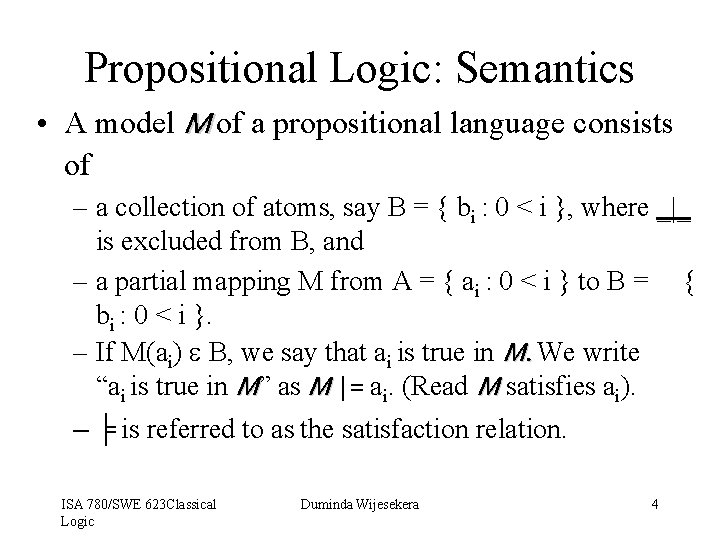Propositional Logic: Semantics • A model M of a propositional language consists of – a collection of atoms, say B = { bi : 0 < i }, where _|_ is excluded from B, and – a partial mapping M from A = { ai : 0 < i } to B = { bi : 0 < i }. – If M(ai) e B, we say that ai is true in M. We write “ai is true in M” as M |= ai. (Read M satisfies ai). – ╞ is referred to as the satisfaction relation. ISA 780/SWE 623 Classical Logic Duminda Wijesekera 4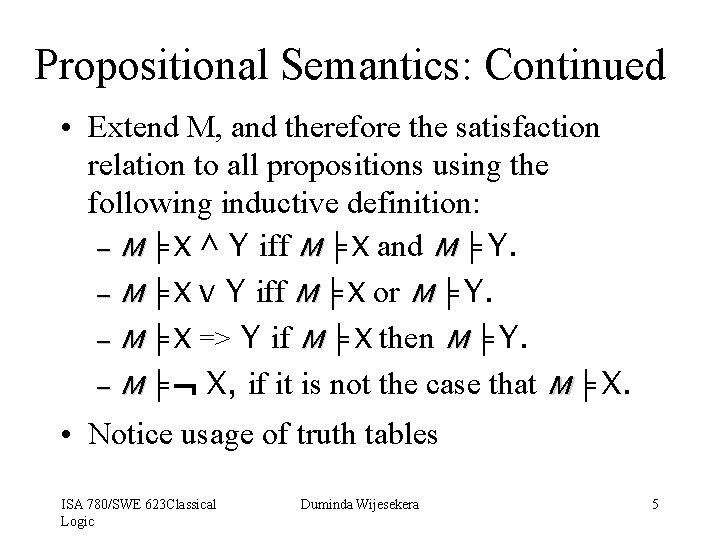Propositional Semantics: Continued • Extend M, and therefore the satisfaction relation to all propositions using the following inductive definition: – M ╞ X ^ Y iff M ╞ X and M ╞ Y. – M ╞ X v Y iff M ╞ X or M ╞ Y. – M ╞ X => Y if M ╞ X then M ╞ Y. – M ╞ X, if it is not the case that M ╞ X. • Notice usage of truth tables ISA 780/SWE 623 Classical Logic Duminda Wijesekera 5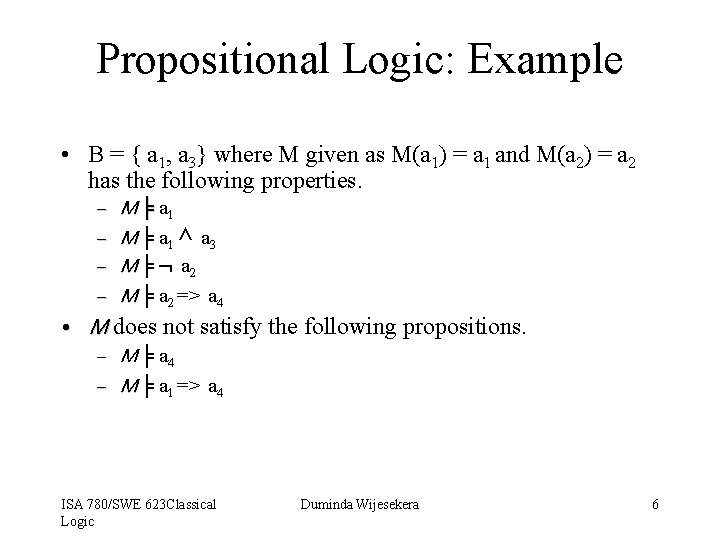Propositional Logic: Example • B = { a 1, a 3} where M given as M(a 1) = a 1 and M(a 2) = a 2 has the following properties. – – M ╞ a 1 ^ a 3 M ╞ a 2 M ╞ a 2 => a 4 • M does not satisfy the following propositions. – M ╞ a 4 – M ╞ a 1 => a 4 ISA 780/SWE 623 Classical Logic Duminda Wijesekera 6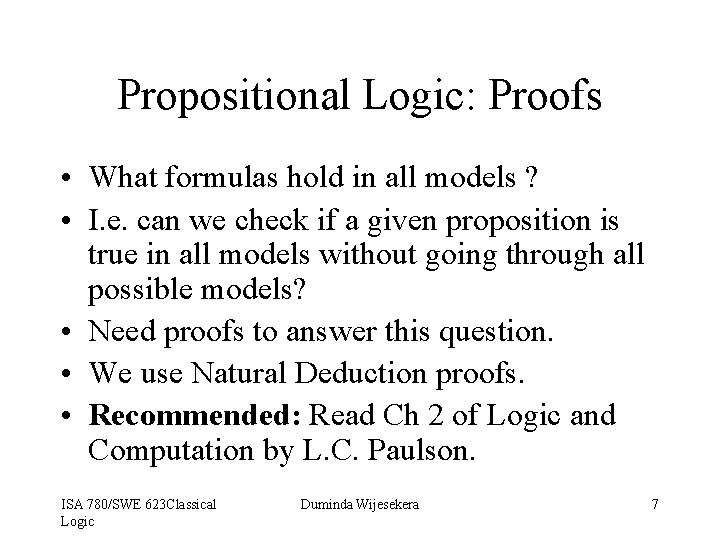Propositional Logic: Proofs • What formulas hold in all models ? • I. e. can we check if a given proposition is true in all models without going through all possible models? • Need proofs to answer this question. • We use Natural Deduction proofs. • Recommended: Read Ch 2 of Logic and Computation by L. C. Paulson. ISA 780/SWE 623 Classical Logic Duminda Wijesekera 7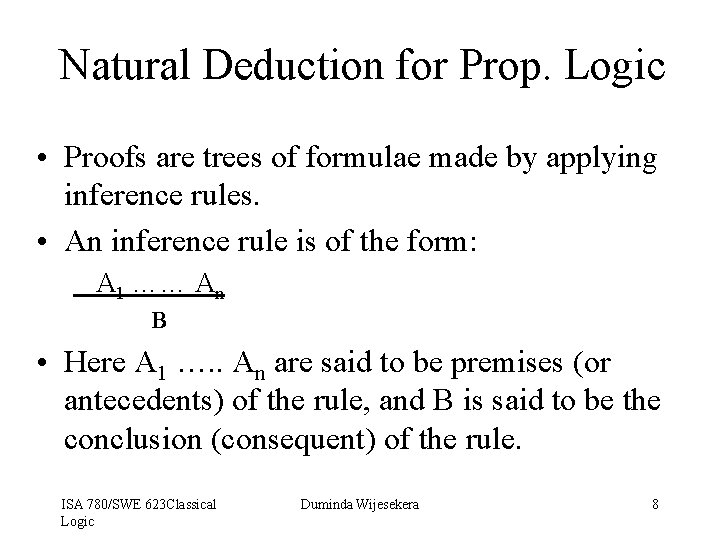Natural Deduction for Prop. Logic • Proofs are trees of formulae made by applying inference rules. • An inference rule is of the form: A 1 …… An B • Here A 1 …. . An are said to be premises (or antecedents) of the rule, and B is said to be the conclusion (consequent) of the rule. ISA 780/SWE 623 Classical Logic Duminda Wijesekera 8Natural Deduction for Prop. Logic • Hence a proofs is a trees whose – Root is theorem to be proved, – Branches are rules, and – Leaves are the assumptions (axioms) of the proof. • Example – A 1 A 2 A 3 C 1 C 2 B 1 B 2 D Assumptions Applications of rules Theorem being proved • There are introduction and elimination rules for each connective in Natural Deduction proof systems. ISA 780/SWE 623 Classical Logic Duminda Wijesekera 9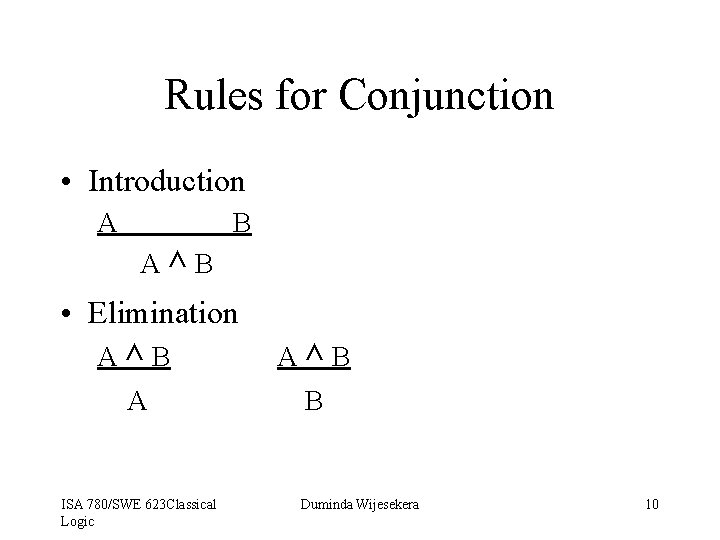Rules for Conjunction • Introduction A B A^B • Elimination A^B A ISA 780/SWE 623 Classical Logic A^B B Duminda Wijesekera 10Rules for Disjunction • Introduction A Av. B B Av. B • Elimination [A] C Av. B [B] C C • [X] denotes discharged assumption X. ISA 780/SWE 623 Classical Logic Duminda Wijesekera 11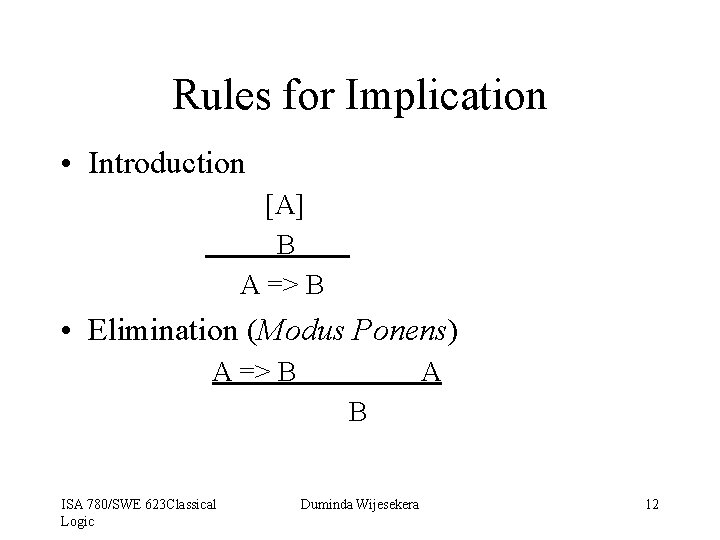Rules for Implication • Introduction [A] B A => B • Elimination (Modus Ponens) A => B A B ISA 780/SWE 623 Classical Logic Duminda Wijesekera 12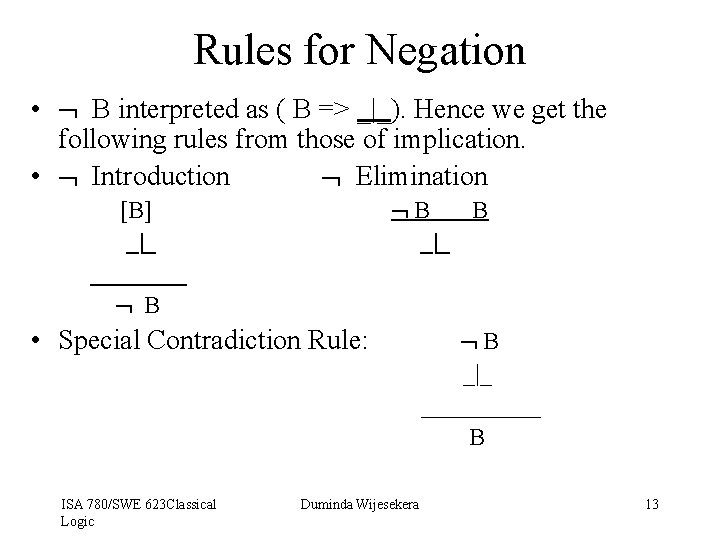Rules for Negation • B interpreted as ( B => _|_). Hence we get the following rules from those of implication. • Introduction Elimination B B _|_ [B] _|_ ____ B • Special Contradiction Rule: ISA 780/SWE 623 Classical Logic Duminda Wijesekera B _|_ _____ B 13Propositional Proofs: Examples • Prove: ( A ^ B ) => (A v B) • Notice: – The outermost connective is =>. Hence, the last step of the proof must be an implication introduction. – That means, we must assume ( A ^ B ) and prove (A v B), and then discharge the assumption by using => introduction rule. – In order to prove (A v B) from ( A ^ B ), we must use v –introduction, and hence must prove either A or B from ( A ^ B ). – This plan forms a skeleton of a proof. ISA 780/SWE 623 Classical Logic Duminda Wijesekera 14Prop. Proof: Example Continued • Prove: ( A ^ B ) => (A v B) [A ^ B ] A Av. B ( A ^ B ) => (A v B) ^ elimination v introduction => introduction • Proofs are analyzed backwards, I. e. start unraveling the logical structure of the conclusion and work backwards to the assumptions. Draw out a plan based on your analysis and write down the formal proof. ISA 780/SWE 623 Classical Logic Duminda Wijesekera 15Derived Rules • These are rules derived from other rules. • Example: A^B B^A • Here is the derivation: A^B B^A B A ^ elimination B^ A ^ introduction ISA 780/SWE 623 Classical Logic Duminda Wijesekera 16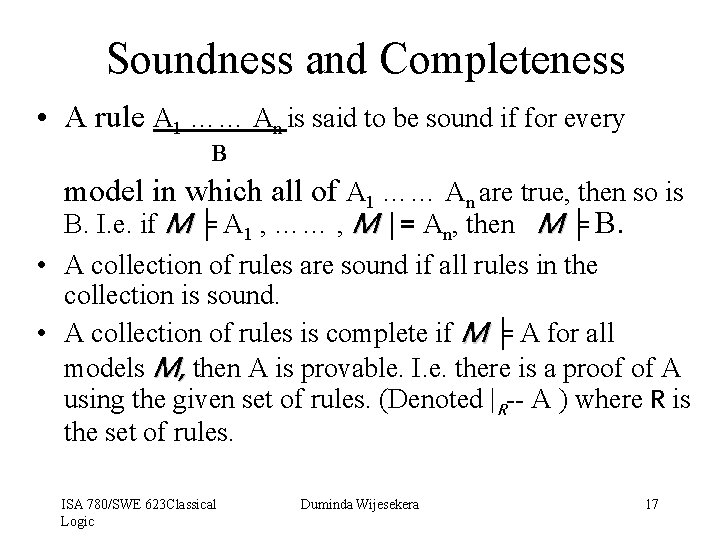Soundness and Completeness • A rule A 1 …… An is said to be sound if for every B model in which all of A 1 …… An are true, then so is B. I. e. if M ╞ A 1 , …… , M |= An, then M ╞ B. • A collection of rules are sound if all rules in the collection is sound. • A collection of rules is complete if M ╞ A for all models M, then A is provable. I. e. there is a proof of A using the given set of rules. (Denoted |R-- A ) where R is the set of rules. ISA 780/SWE 623 Classical Logic Duminda Wijesekera 17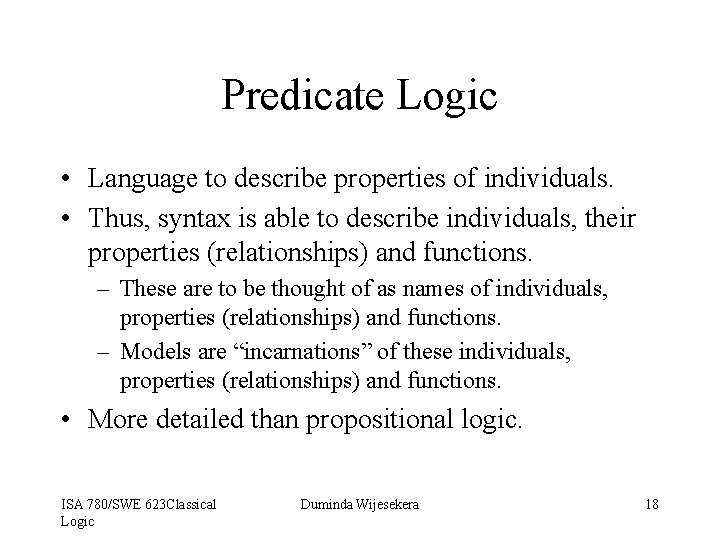Predicate Logic • Language to describe properties of individuals. • Thus, syntax is able to describe individuals, their properties (relationships) and functions. – These are to be thought of as names of individuals, properties (relationships) and functions. – Models are “incarnations” of these individuals, properties (relationships) and functions. • More detailed than propositional logic. ISA 780/SWE 623 Classical Logic Duminda Wijesekera 18Predicate Logic: Syntax • A collection of constants– say { ci : i >= 0 }. – Constants are names for individuals. E. g. : 0, 1. – Note: not all individuals in a model have names. • A collection of variables– say { xi : i >= 0 }. – Needed to generically refer to individuals. – Think of them as standing in place of pronouns like it, she. • A collection of function symbols- say { fi : i >= 0 }. – May be of different arities, and may be typed. E. g. : +(x, y) • A collection of predicate symbols- say { pi : i >= 0 }. – May be of different arities. – Encodes properties of individuals. E. g. : prime(x). ISA 780/SWE 623 Classical Logic Duminda Wijesekera 19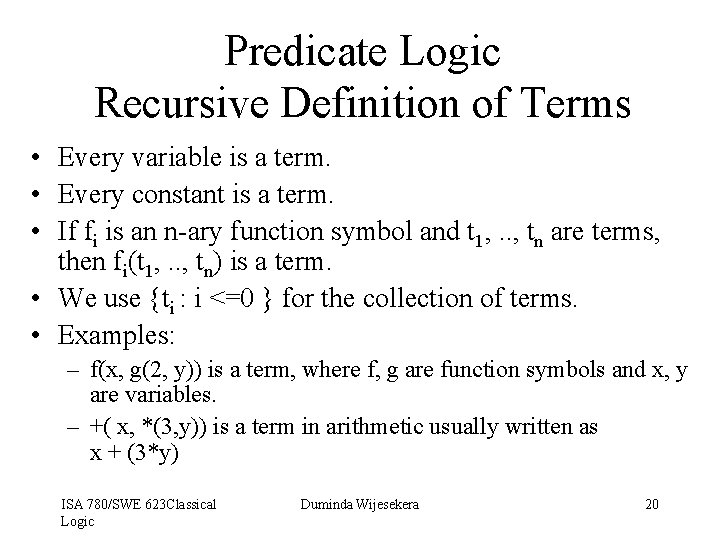Predicate Logic Recursive Definition of Terms • Every variable is a term. • Every constant is a term. • If fi is an n-ary function symbol and t 1, . . , tn are terms, then fi(t 1, . . , tn) is a term. • We use {ti : i <=0 } for the collection of terms. • Examples: – f(x, g(2, y)) is a term, where f, g are function symbols and x, y are variables. – +( x, *(3, y)) is a term in arithmetic usually written as x + (3*y) ISA 780/SWE 623 Classical Logic Duminda Wijesekera 20Recursive Definition of Formulas • If pi is an n-ary predicate symbol and t 1, . . , tn are terms, then pi(t 1, . . , tn ) is an atomic formula. • If A and B are formulas, then so are: – A ^ B, A v B, A, A => B. – xi A(xi), where xi is a variable. • , are referred to as the universal and existential quantifier, respectively. • A formula that does not have either quantifier is said to be a quantifier free. ISA 780/SWE 623 Classical Logic Duminda Wijesekera 21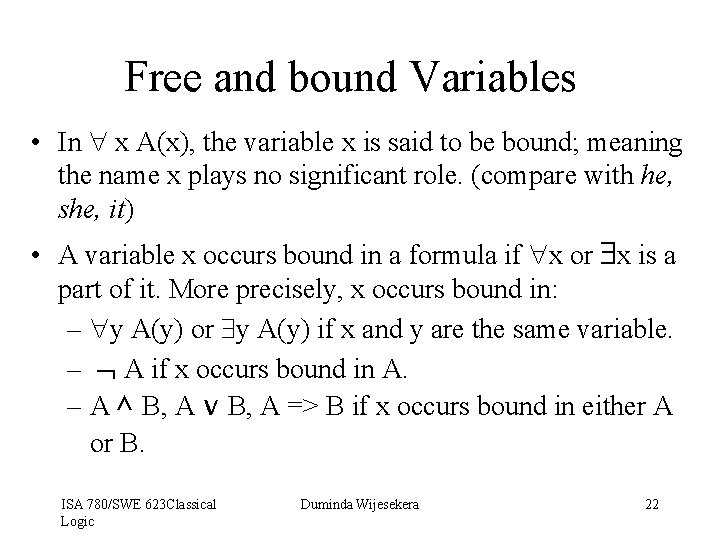Free and bound Variables • In x A(x), the variable x is said to be bound; meaning the name x plays no significant role. (compare with he, she, it) • A variable x occurs bound in a formula if x or x is a part of it. More precisely, x occurs bound in: – y A(y) or y A(y) if x and y are the same variable. – A if x occurs bound in A. – A ^ B, A v B, A => B if x occurs bound in either A or B. ISA 780/SWE 623 Classical Logic Duminda Wijesekera 22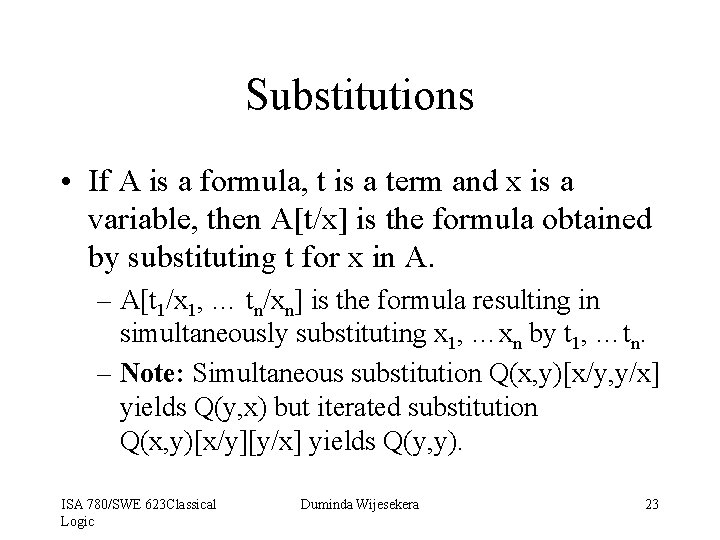Substitutions • If A is a formula, t is a term and x is a variable, then A[t/x] is the formula obtained by substituting t for x in A. – A[t 1/x 1, … tn/xn] is the formula resulting in simultaneously substituting x 1, …xn by t 1, …tn. – Note: Simultaneous substitution Q(x, y)[x/y, y/x] yields Q(y, x) but iterated substitution Q(x, y)[x/y][y/x] yields Q(y, y). ISA 780/SWE 623 Classical Logic Duminda Wijesekera 23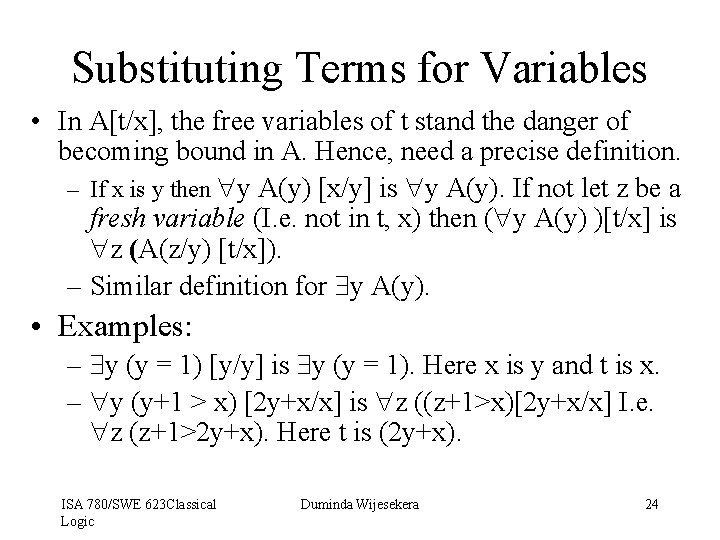Substituting Terms for Variables • In A[t/x], the free variables of t stand the danger of becoming bound in A. Hence, need a precise definition. – If x is y then y A(y) [x/y] is y A(y). If not let z be a fresh variable (I. e. not in t, x) then ( y A(y) )[t/x] is z (A(z/y) [t/x]). – Similar definition for y A(y). • Examples: – y (y = 1) [y/y] is y (y = 1). Here x is y and t is x. – y (y+1 > x) [2 y+x/x] is z ((z+1>x)[2 y+x/x] I. e. z (z+1>2 y+x). Here t is (2 y+x). ISA 780/SWE 623 Classical Logic Duminda Wijesekera 24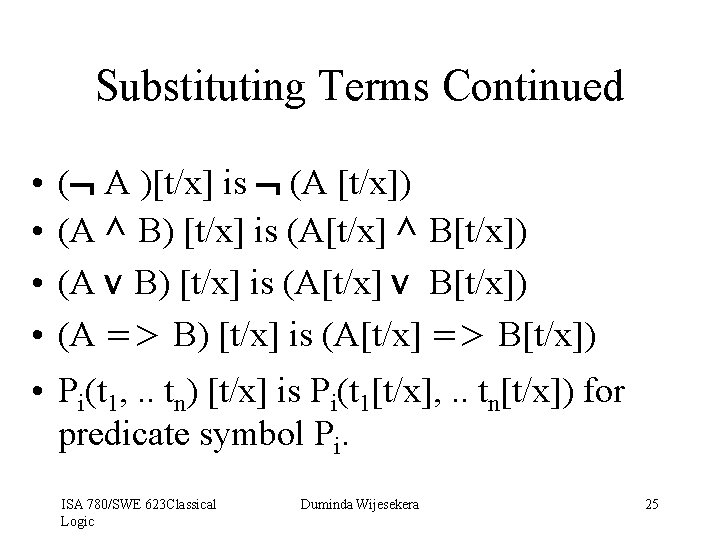Substituting Terms Continued • • ( A )[t/x] is (A [t/x]) (A ^ B) [t/x] is (A[t/x] ^ B[t/x]) (A v B) [t/x] is (A[t/x] v B[t/x]) (A => B) [t/x] is (A[t/x] => B[t/x]) • Pi(t 1, . . tn) [t/x] is Pi(t 1[t/x], . . tn[t/x]) for predicate symbol Pi. ISA 780/SWE 623 Classical Logic Duminda Wijesekera 25Predicate Logic: Semantics • A model consists of – A set (of individuals), say A = { ai : i >= 0 }. – A set of total functions Fn = { fni : i >= 0 } on A. • I. e. fni(aj) is some ak for every aj. – A set of predicates Pr = { pri : i >= 0 } over A. • Do not have to be total. • Can have many arities. ISA 780/SWE 623 Classical Logic Duminda Wijesekera 26Interpreting Syntax • Mapping from Syntax to Semantics: – A mapping m. Cons : { ci : I >= 0 } to A={ai: i >= 0}. • Need not be ONTO A. I. e. there could be unnamed individuals in the semantic domain. – A mapping m. Fun : { fi : I >= 0 } to Fn={fni: i >= 0}. • Need not be onto. I. e. there could be unnamed functions in the semantic domain. – A mapping m. Pred: { pi : I >= 0 } to Pr={pri: i >= 0}. • Need not be onto. I. e. there could be unnamed predicates in the semantic domain. ISA 780/SWE 623 Classical Logic Duminda Wijesekera 27Interpreting Formulas: naming • We do not interpret formulas with free variables. • In order to interpret quantified formulas, need to expand the syntax by adding a constant in the syntax for each unnamed individual in the model. – I. e. for each ai for which there is no cj such that Fn(cj ) is ai, add a new constant Cai to the syntax. – Now expand the definition of terms to include these new constants. Let new. T = { Nti : i >= 0} be the collection of new terms so defined. ISA 780/SWE 623 Classical Logic Duminda Wijesekera 28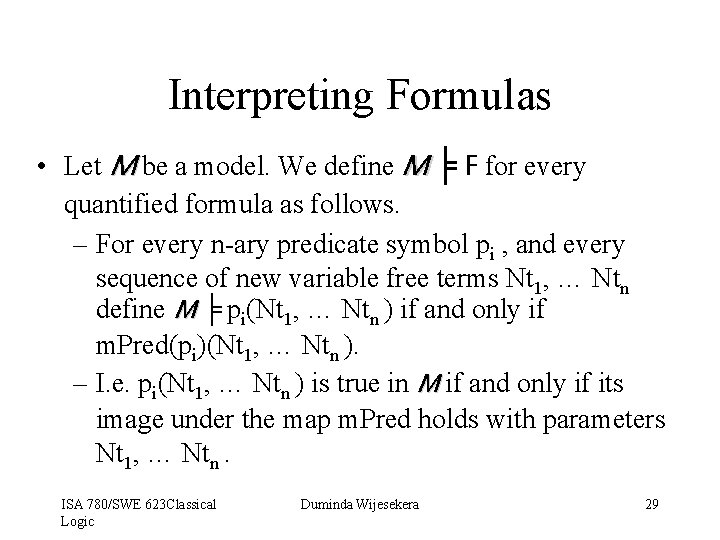Interpreting Formulas • Let M be a model. We define M ╞ F for every quantified formula as follows. – For every n-ary predicate symbol pi , and every sequence of new variable free terms Nt 1, … Ntn define M ╞ pi(Nt 1, … Ntn ) if and only if m. Pred(pi)(Nt 1, … Ntn ). – I. e. pi(Nt 1, … Ntn ) is true in M if and only if its image under the map m. Pred holds with parameters Nt 1, … Ntn. ISA 780/SWE 623 Classical Logic Duminda Wijesekera 29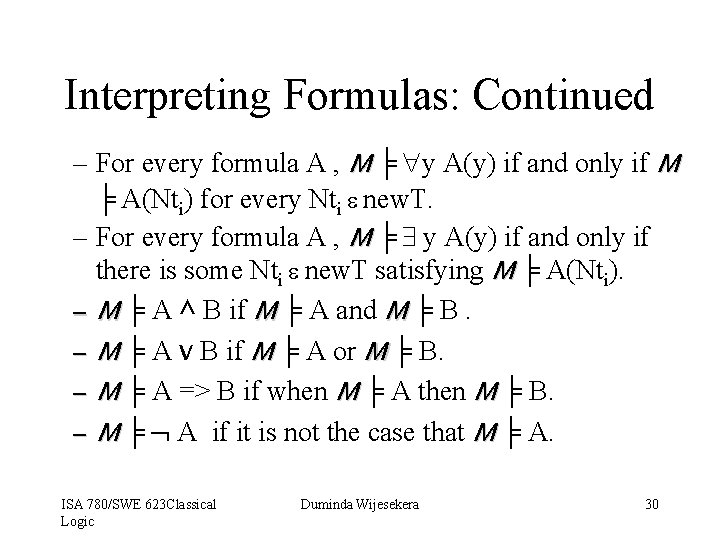Interpreting Formulas: Continued – For every formula A , M ╞ y A(y) if and only if M ╞ A(Nti) for every Nti e new. T. – For every formula A , M ╞ y A(y) if and only if there is some Nti e new. T satisfying M ╞ A(Nti). – M ╞ A ^ B if M ╞ A and M ╞ B. – M ╞ A v B if M ╞ A or M ╞ B. – M ╞ A => B if when M ╞ A then M ╞ B. – M ╞ A if it is not the case that M ╞ A. ISA 780/SWE 623 Classical Logic Duminda Wijesekera 30Proof Rules for Predicate Logic • Proof rules of introduction and elimination of ^, v, =>, and . • New rules required for introduction and elimination of and quantifiers. ISA 780/SWE 623 Classical Logic Duminda Wijesekera 31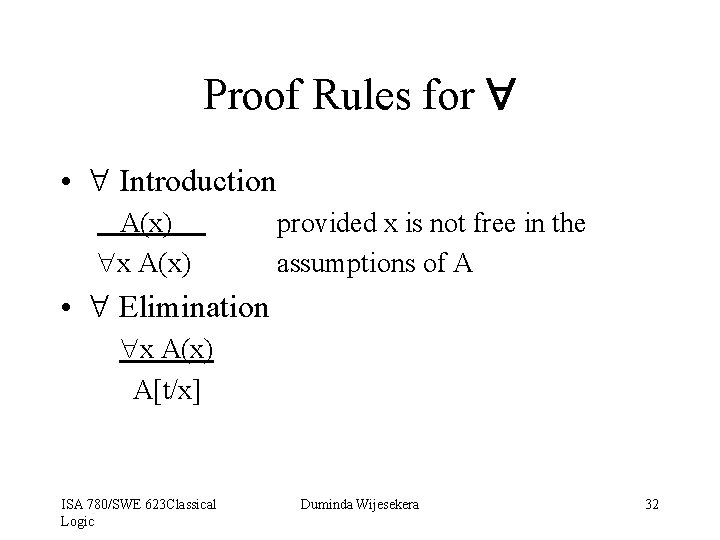Proof Rules for • Introduction A(x) x A(x) provided x is not free in the assumptions of A • Elimination x A(x) A[t/x] ISA 780/SWE 623 Classical Logic Duminda Wijesekera 32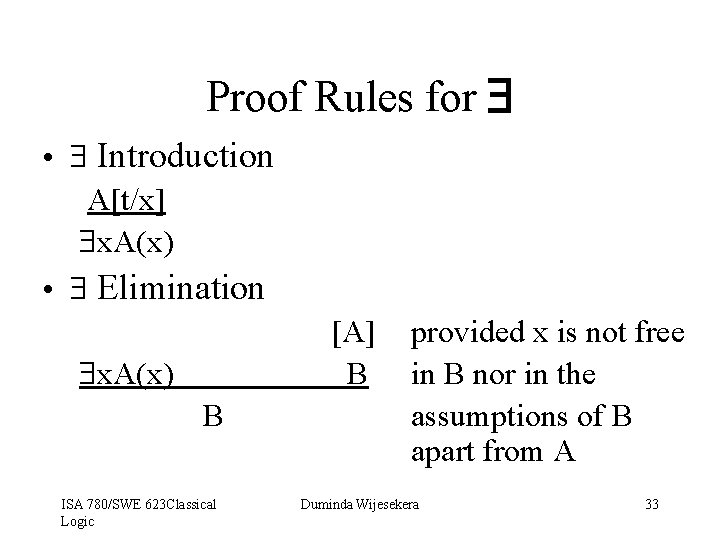Proof Rules for • Introduction A[t/x] x. A(x) • Elimination [A] B x. A(x) B ISA 780/SWE 623 Classical Logic provided x is not free in B nor in the assumptions of B apart from A Duminda Wijesekera 33An Example Proof • Prove (( x A(x)) ^ B) => ( x (A(x)^ B)) provided that x is not free in B. • Plan: – Since outer connective is =>, need to use => introduction at the last step. Hence can use ( x A(x)) ^ B as an assumption for the steps above. – Now in order to get x (A(x)^ B) using introduction, we need to get A[t/x] )^ B. – Can use ^ elimination to ( x A(x)) ^ B and obtain B – Can use x elimination to get A[t/x]. ISA 780/SWE 623 Classical Logic Duminda Wijesekera 34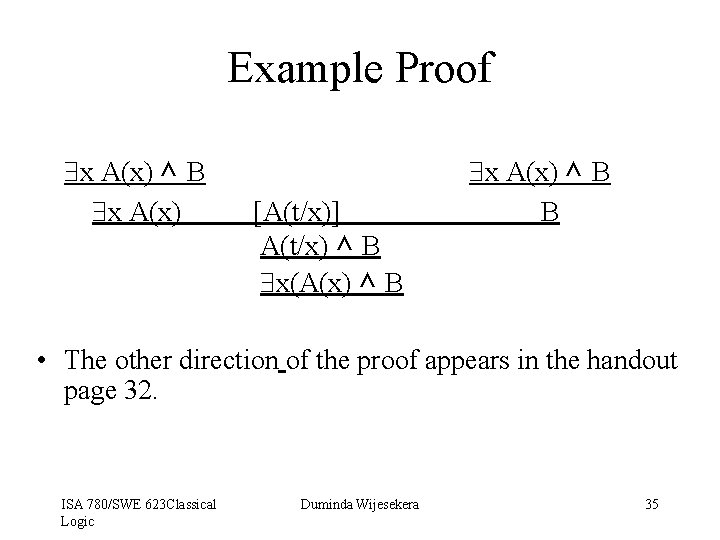Example Proof x A(x) ^ B x A(x) [A(t/x)] A(t/x) ^ B x(A(x) ^ B x A(x) ^ B B • The other direction of the proof appears in the handout page 32. ISA 780/SWE 623 Classical Logic Duminda Wijesekera 35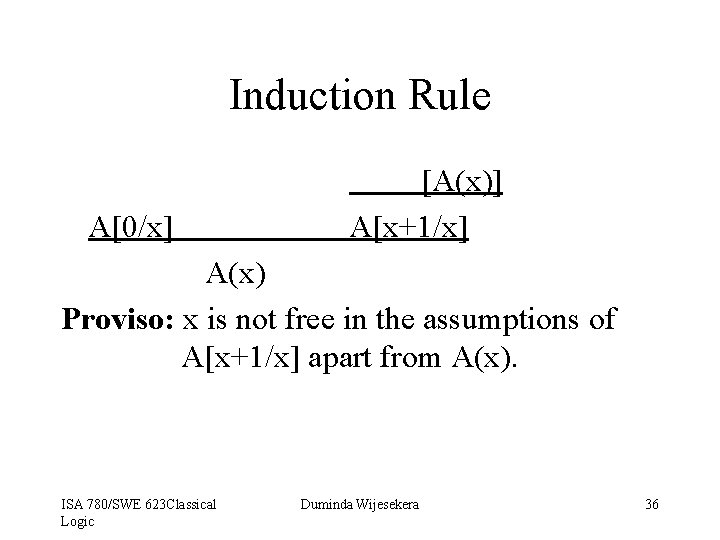Induction Rule A[0/x] [A(x)] A[x+1/x] A(x) Proviso: x is not free in the assumptions of A[x+1/x] apart from A(x). ISA 780/SWE 623 Classical Logic Duminda Wijesekera 36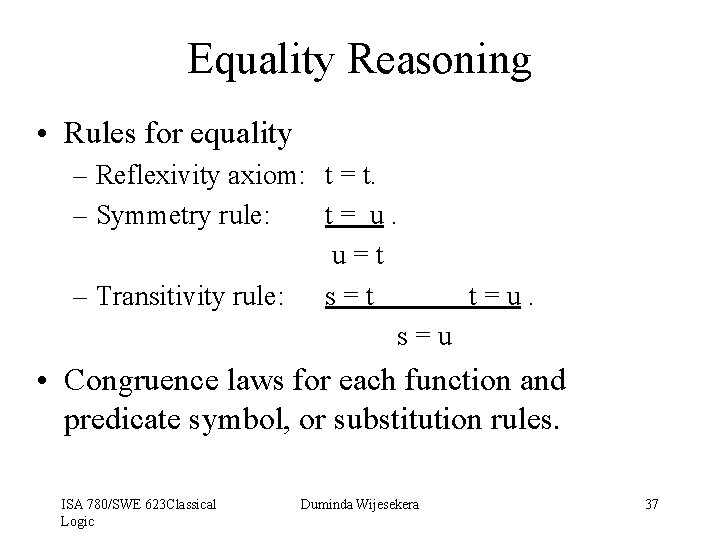Equality Reasoning • Rules for equality – Reflexivity axiom: t = t. – Symmetry rule: t= u. u=t – Transitivity rule: s = t t=u. s=u • Congruence laws for each function and predicate symbol, or substitution rules. ISA 780/SWE 623 Classical Logic Duminda Wijesekera 37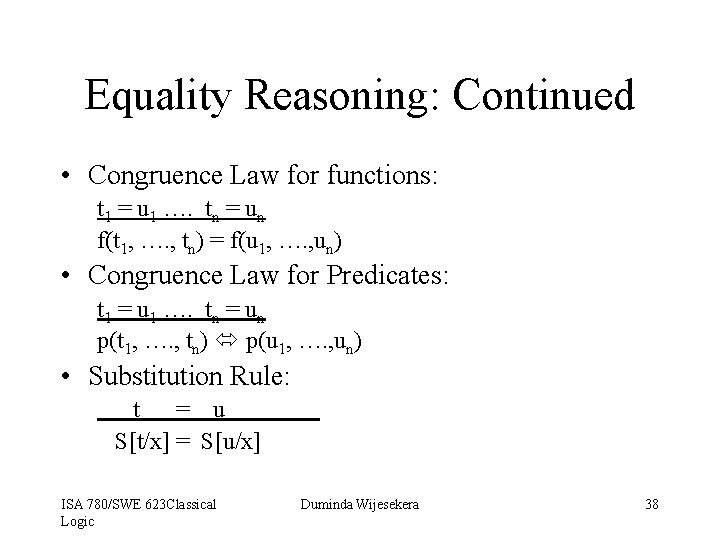Equality Reasoning: Continued • Congruence Law for functions: t 1 = u 1 …. tn = un f(t 1, …. , tn) = f(u 1, …. , un) • Congruence Law for Predicates: t 1 = u 1 …. tn = un p(t 1, …. , tn) p(u 1, …. , un) • Substitution Rule: t = u S[t/x] = S[u/x] ISA 780/SWE 623 Classical Logic Duminda Wijesekera 38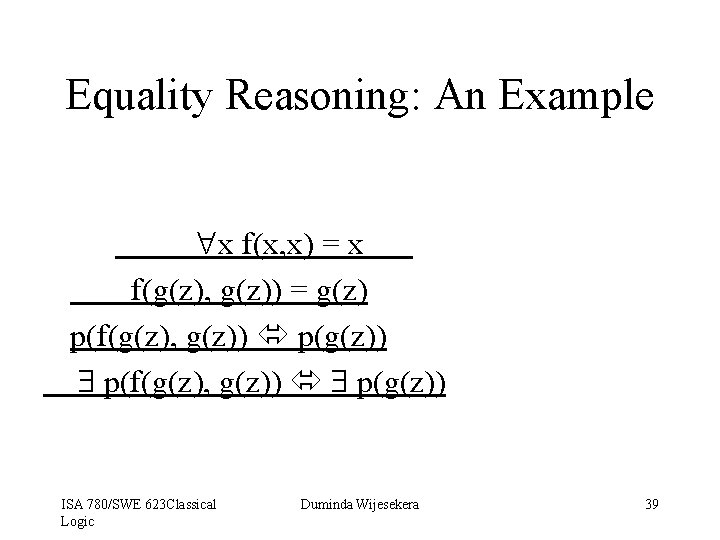Equality Reasoning: An Example x f(x, x) = x f(g(z), g(z)) = g(z) p(f(g(z), g(z)) p(g(z)) ISA 780/SWE 623 Classical Logic Duminda Wijesekera 39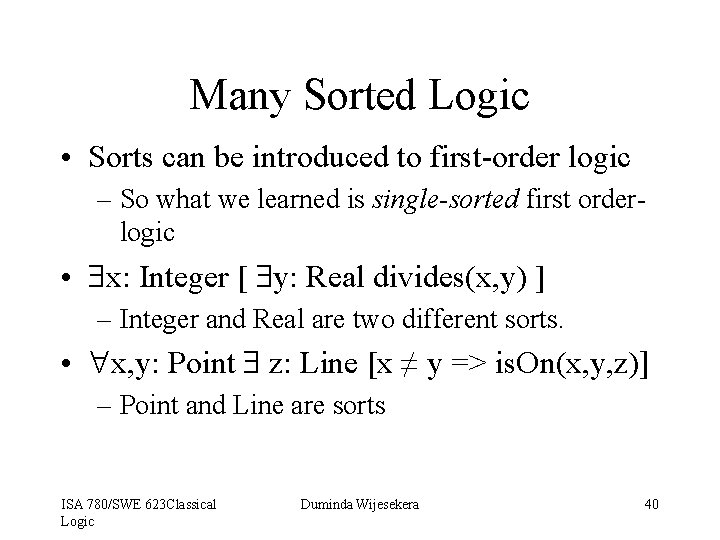Many Sorted Logic • Sorts can be introduced to first-order logic – So what we learned is single-sorted first orderlogic • x: Integer [ y: Real divides(x, y) ] – Integer and Real are two different sorts. • x, y: Point z: Line [x ≠ y => is. On(x, y, z)] – Point and Line are sorts ISA 780/SWE 623 Classical Logic Duminda Wijesekera 40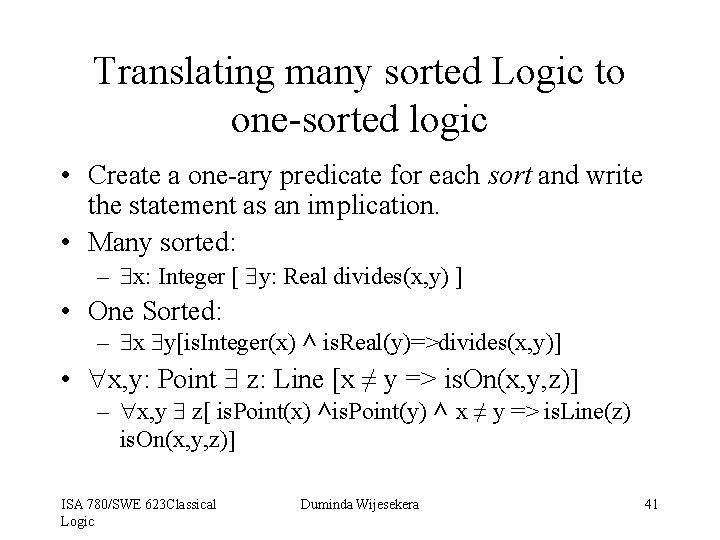Translating many sorted Logic to one-sorted logic • Create a one-ary predicate for each sort and write the statement as an implication. • Many sorted: – x: Integer [ y: Real divides(x, y) ] • One Sorted: – x y[is. Integer(x) ^ is. Real(y)=>divides(x, y)] • x, y: Point z: Line [x ≠ y => is. On(x, y, z)] – x, y z[ is. Point(x) ^is. Point(y) ^ x ≠ y => is. Line(z) is. On(x, y, z)] ISA 780/SWE 623 Classical Logic Duminda Wijesekera 41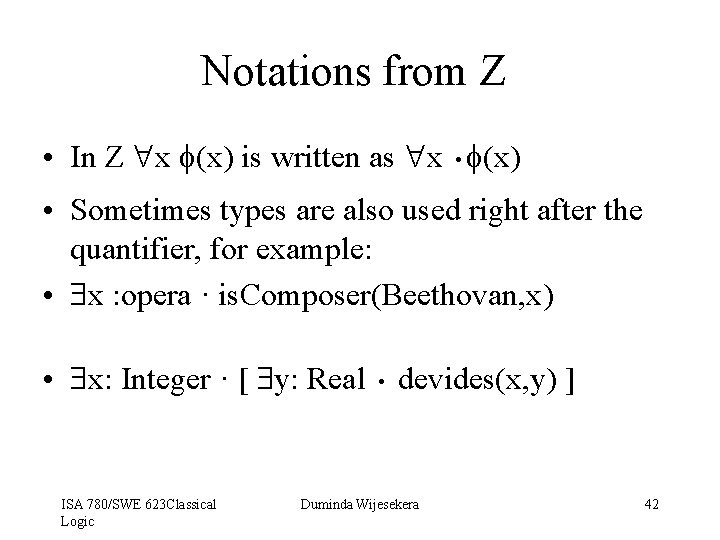Notations from Z . • In Z x f(x) is written as x f(x) • Sometimes types are also used right after the quantifier, for example: • x : opera · is. Composer(Beethovan, x) . • x: Integer · [ y: Real devides(x, y) ] ISA 780/SWE 623 Classical Logic Duminda Wijesekera 42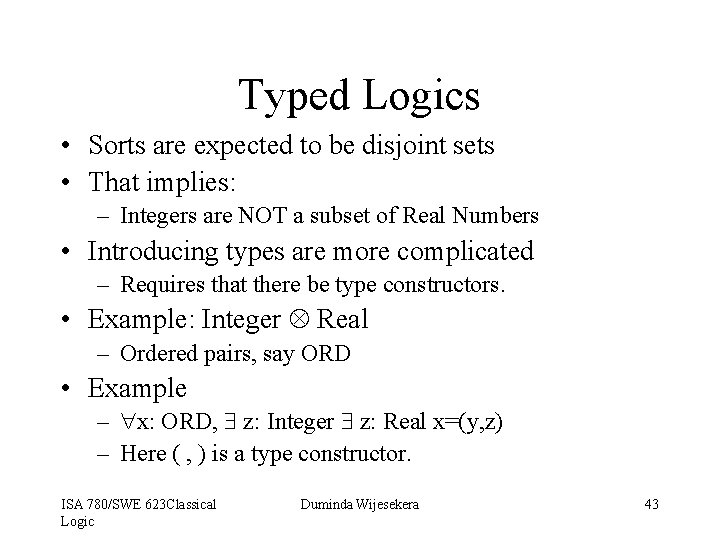Typed Logics • Sorts are expected to be disjoint sets • That implies: – Integers are NOT a subset of Real Numbers • Introducing types are more complicated – Requires that there be type constructors. • Example: Integer Real – Ordered pairs, say ORD • Example – x: ORD, z: Integer z: Real x=(y, z) – Here ( , ) is a type constructor. ISA 780/SWE 623 Classical Logic Duminda Wijesekera 43Why is this an Issue? • • • How to prove: (x, y) = (a, b) iff x=a and y=b Similar problems occur with sets, lists etc. What is the problem? The equality theory has to be built. Sets create more problems: – Set quantifiers are problematic – Leads to FULL second order logic • No complete Σ 1 proof theory ISA 780/SWE 623 Classical Logic Duminda Wijesekera 44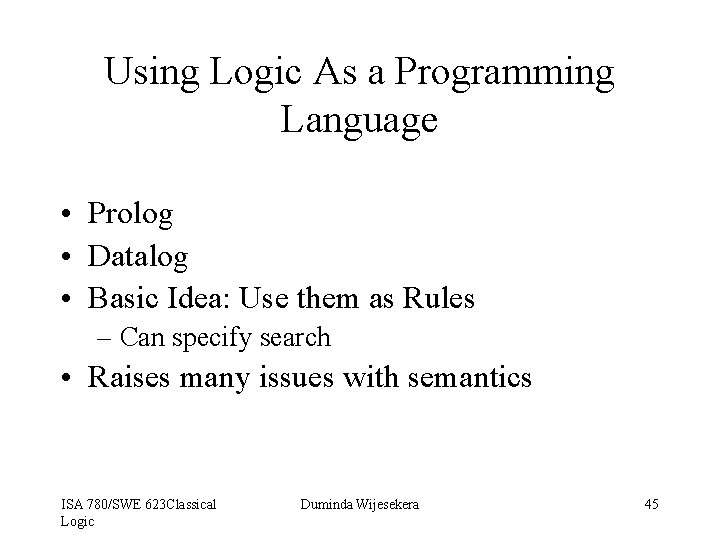Using Logic As a Programming Language • Prolog • Datalog • Basic Idea: Use them as Rules – Can specify search • Raises many issues with semantics ISA 780/SWE 623 Classical Logic Duminda Wijesekera 45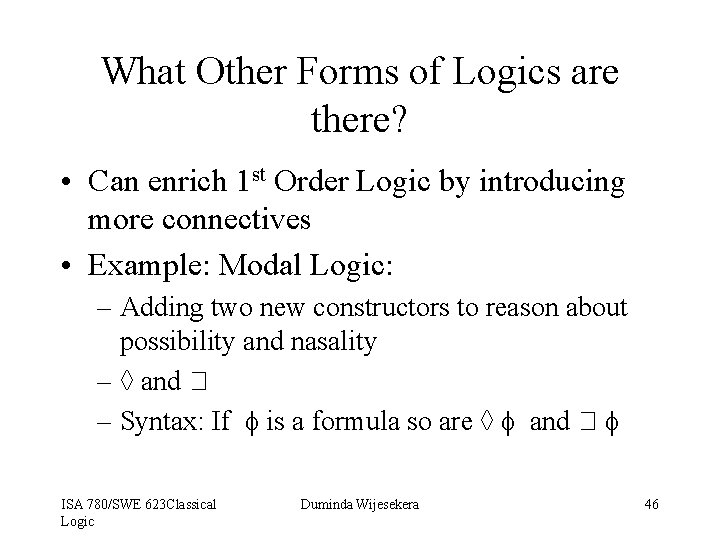What Other Forms of Logics are there? • Can enrich 1 st Order Logic by introducing more connectives • Example: Modal Logic: – Adding two new constructors to reason about possibility and nasality – ◊ and – Syntax: If f is a formula so are ◊ f and f ISA 780/SWE 623 Classical Logic Duminda Wijesekera 46Applications of Modal Logic • • • Dynamic Logic Temporal Logics Theories of Motion Theories of Change Theories of Knowledge Applications in security – Access control – Delegation • See separate transparency on Modal, Dynamic and Temporal Logics ISA 780/SWE 623 Classical Logic Duminda Wijesekera 47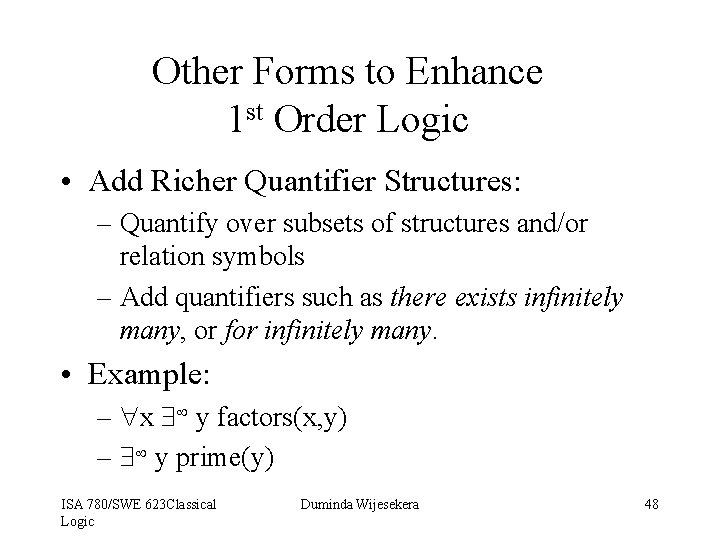Other Forms to Enhance 1 st Order Logic • Add Richer Quantifier Structures: – Quantify over subsets of structures and/or relation symbols – Add quantifiers such as there exists infinitely many, or for infinitely many. • Example: – x y factors(x, y) – y prime(y) ISA 780/SWE 623 Classical Logic Duminda Wijesekera 48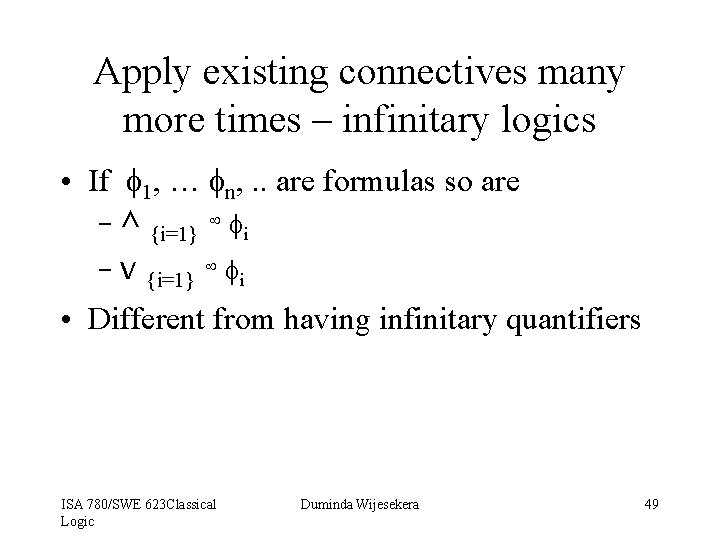Apply existing connectives many more times – infinitary logics • If f 1, … fn, . . are formulas so are – ^ {i=1} fi – v {i=1} fi • Different from having infinitary quantifiers ISA 780/SWE 623 Classical Logic Duminda Wijesekera 49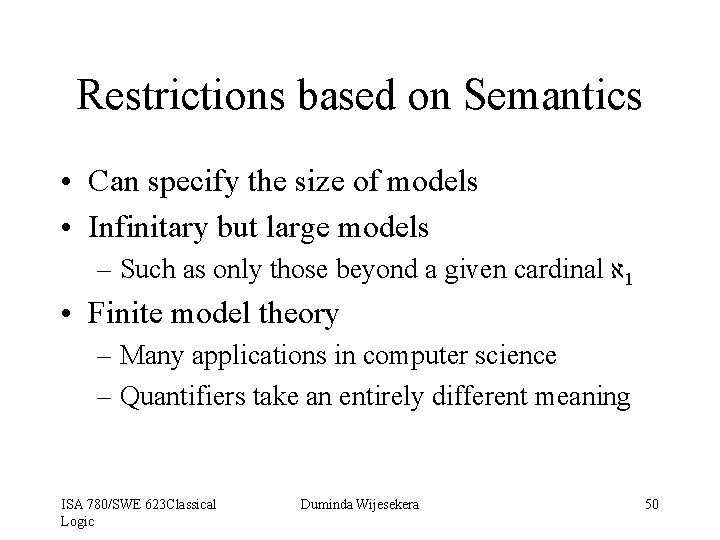Restrictions based on Semantics • Can specify the size of models • Infinitary but large models – Such as only those beyond a given cardinal א 1 • Finite model theory – Many applications in computer science – Quantifiers take an entirely different meaning ISA 780/SWE 623 Classical Logic Duminda Wijesekera 50Those that reject basic assumptions of Classical Logic • Intuitionism • Why is (A → B) v (B → A) a tautology ? – Pigenhole principle in classical truth values • Similar argument for (A 1 → A 2) v (A 2 → A 3) …. (An → An+1) v (An+1 → A 1) • Consequence: P v P does not have to be true! • Infinitely many truth values • Leads to Kripke Semantics with worlds with increasing truth values • Used in the semantics of programming languages and type theories ISA 780/SWE 623 Classical Logic Duminda Wijesekera 51